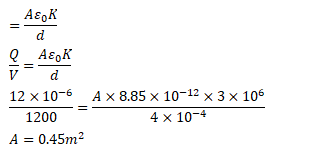# An air filled parallel-plate capacitor is to be constructedQuestion:

An air filled parallel-plate capacitor is to be constructed which can store $12 \mu \mathrm{C}$ of charge when operated at $1200 \mathrm{~V}$. What can be the minimum plate area of the capacitor? The dielectric strength of air $3 \times 10^{6} \mathrm{~V} / \mathrm{m}$.

Solution: